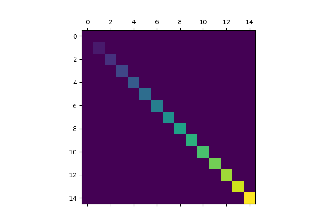# matplotlib.axes.Axes.matshow¶

Axes.matshow(self, Z, **kwargs)[source]

Plot the values of a 2D matrix or array as color-coded image.

The matrix will be shown the way it would be printed, with the first row at the top. Row and column numbering is zero-based.

Parameters: Zarray-like(M, N)The matrix to be displayed. AxesImage **kwargsimshow arguments

imshow
More general function to plot data on a 2D regular raster.

Notes

This is just a convenience function wrapping imshow to set useful defaults for displaying a matrix. In particular:

• Set origin='upper'.
• Set interpolation='nearest'.
• Set aspect='equal'.
• Ticks are placed to the left and above.
• Ticks are formatted to show integer indices.

## Examples using matplotlib.axes.Axes.matshow¶